Reach Us+44-1522-440391
Regenerator Analysis with Finite Elements | OMICS International
Journal of Fundamentals of Renewable Energy and Applications
All submissions of the EM system will be redirected to Online Manuscript Submission System. Authors are requested to submit articles directly to Online Manuscript Submission System of respective journal.

# Regenerator Analysis with Finite Elements

Rauno Holopainen1* and Eero-Matti Salonen2

1Finnish Institute of Occupational Health, Developing Indoor Environments, Finland

2Aalto University, School of Engineering, Department of Civil and Structural Engineering, Finland

Corresponding Author:
Rauno Holopainen
Developing Indoor Environments
Finnish Institute of Occupational Health
Arinatie 3 A, FIN-00370 Helsinki, Finland
Tel: +358 43 825 2914
Fax: +358 9 506 1087
E-mail: [email protected]

Received date: May 14, 2015; Accepted date: June 07, 2015; Published date: June 14, 2015

Citation: Holopainen R, Salonen EM (2015) Regenerator Analysis with Finite Elements. J Fundam Renewable Energy Appl 5:173. doi: 10.4173/20904541.1000173

Copyright: © 2015 Holopainen R , et al. This is an open-access article distributed under the terms of the Creative Commons Attribution License, which permits unrestricted use, distribution, and reproduction in any medium, provided the original author and source are credited.

Visit for more related articles at Journal of Fundamentals of Renewable Energy and Applications

#### Abstract

The classic one-dimensional regenerator problem has been solved numerically in a new way. Instead of marching in time, the hot and cold periods are treated as boundary value problems in rectangular domains. To solve the boundary value problems, a space-time finite element method using the commercial package COMSOL 4.3 with MATLAB with a Galerkin formulation has been applied. This approach minimizes the coding effort. The code solves a problem automatically with three consecutive meshes of increasing densities. We present results of three example cases in some detail.

#### Keywords

Regenerator; COMSOL; MATLAB

#### Nomenclature

Latin symbols

A    total heat transfer surface area (m2)

c    specific heat at constant pressure (kj/kgk )

er    relative error estimate ( − )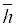overall heat transfer coefficient (w/m2k)

L    length of storage unit (m )

m   mass (kg )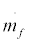mass rate of fluid flow

P    period time length (s )

r     effective convergence rate ( − )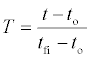dimensionless temperature ( − )

t    temperature (°C ,k )

x    axial coordinate (m)

Greek symbols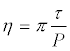nondimensional time coordinate ( − )

ηREG thermal ratio ( − )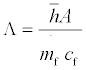reduced length ( − )

λ grid refinement ratio ( − )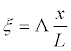nondimensional position coordinate ( − )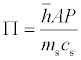reduced period ( − )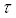time (s)

Subscripts

c  coarse

f   fluid, fine

i      inlet

m     medium

s   solid (storage) material

o   initial

Superscripts

'     Hot period

"     Cold period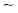Space average

#### Introduction

Heat recovery is a topical issue, as it has a major effect on buildings’ energy efficiency. A typical heat recovery application is a ventilation system, in which intake air is heated by warm outlet air. This article focuses on regenerative heat exchanger computational modelling, in which mass is alternately heated and cooled by changing the direction of the air flow.

Classic one-dimensional counter flow regenerator analysis demands normally the use of numerical methods. In this article, the analysis is performed in a new way by applying the finite element method. This approach has certain advantages from the coding effort and accuracy perspectives. We are not aware of the use of the finite element method as a pure boundary value problem in this connection.

We have based our presentation strongly on the theory and arguments given in Schmidt and Willmott . The same theme has been treated, for example, in a rather recent text on regenerative heat transfer in Willmott . However, this latter reference contains no essential changes with respect to the theme of the present article. We have preferred mostly the notations of Schmidt and Willmott .

Basic Equation

As mentioned, we will follow the theory given in Schmidt and Willmot . We first consider the basic equations in the single blow case. The regenerator case – to be described in Section 3 – can then be seen as consisting of more or less of consecutive single blow cases.

The setting is briefly illustrated in Figure 1a. The fluid and solid temperatures to be determined tf and ts depend on device axial position x and time τ. As the initial condition, ts is given on boundary τ = 0 and as the boundary condition, tf is given on fluid inflow boundary x = 0.

Figure 1: (a) Solution domain in the dimensional x,Τ -space and (b) in the corresponding dimensionless ζ , η-space

Schmidt and Willmott  describes the governing energy balance equations and the procedures for transforming the equations into dimensionless forms, as indicated in Figure 1b. The dimensionless fluid and solid temperatures are Tf and Ts and these depend on the dimensionless position coordinate ξ and dimensionless time η. The dimensionless length measures corresponding to L and P in Figure 1a are ٨ and Π. The mathematical treatment of the problem takes place most conveniently in the dimensionless form.

The governing field equations are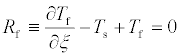(1)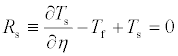(2)

Notations R refer to field equation residuals. This terminology is common in the finite element method literature – e.g. (Zienkiewicz and Taylor  – and here means that certain formulas to follow can be written in a short form.

The initial and boundary conditions become, respectively:

Ts (ξ, 0) = 0  (3)

Tf (0, η) = 1  (4)

The standard numerical approach used in e.g. (Schmidt and Willmott  is based on the finite difference method. Furthermore, the time coordinate is also taken into account in Schmidt and Willmott  in the standard way by “marching” in the time direction in a stepwise manner.

The numerical approach employed in this article is based on the finite element method. In addition, the space and time coordinates are treated equally. Considering the very similar roles of ξ and η in equations (1) and (2), this seems appropriate. So no marching in time is performed. The case shown in Figure 1b is considered as just as a boundary value problem, with boundary conditions (3) and (4) on the two boundary parts η = 0 and ξ = 0, respectively. Thus, we now also alternatively call (3) a boundary condition.

As is well known, the finite element method is based on integral type presentations with the method of weighted residuals. The most common versions of the method of weighted residuals are the Galerkin method and the least-squares method. We experimented with two Galerkin type versions (Formulations I and II) and with a least-squares

version (Formulation III). The weak forms corresponding to these formulations are listed below

Formulation I the weak form is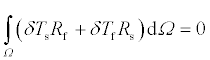(5)

Formulation II The weak form is(6)

Formulation III The weak form is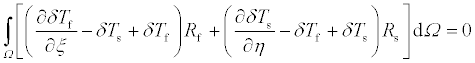(7)

The integrals are over domain Ω= (0,Λ) × (0,Π) in Figure 1b. The Galerkin method is normally defined as one in which the same basis functions used in the approximations of the unknowns are employed as weighting functions. This can also be interpreted so that the weighting functions (before discretization) are variations (δTf, δTs ) of the unknown functions (Tf,Ts ). The two options for choosing the weighting functions are seen in Formulations I and II. The least-squares version is based on the functional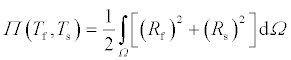(8)

Requiring the functional to have a stationary value produces the weak form (7).

The use of the finite element method may be justified by the fact that certain general shelf software – especially COMSOL Multi-physics with the finite element method – allows the applier to feed his/her problem directly as a weak form. We used this option. Obviously, this results in the required coding effort becoming rather small.

Because of the simple rectangular solution domain, uniform meshes consisting of four-node rectangular Lagrangian elements were used for both unknowns Tf and Ts.

Figure 2 shows crude meshes for two geometries considered below. It should be noted, however, that the elements are not quite standard, as they are actually space-time elements. Boundary conditions (3) and (4) are satisfied by giving the corresponding nodal values.

In the generated MATLAB-code used in connection with COMSOL Multi-physics, care was taken to gain confidence in the accuracy of the numerical results. We more or less followed the recommendations of references Roache . Sinclair et al.  in every calculation case, we used three meshes of increasing densities: coarse, medium and fine, and we use the corresponding subscripts c, m and f respectively when referring to the appropriate values. If hc is the typical mesh size of a coarse mesh, the corresponding mesh sizes for the medium and the fine meshes are hm = hc⁄λ and hf = hc⁄λ2 , respectively, where λ is the refinement ratio or the scale factor . We employ the value λ=2.

The effective convergence rate r for quantity f is obtained from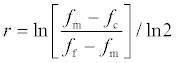(9)

Following (Roache, 2009), the relative error estimate expression er for result f with the finest mesh is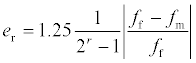(10)

where the multiplier 1.25 is a “factor of safety”. Use of the Richardson extrapolation was not considered necessary.

The case sketched in Figure 2a is taken from Example 2.1 in Schmidt and Willmott  and the case in Figure 2b is the same as the first case but with the domain measures switched. The crude meshes shown in the figures are selected so that the elements are roughly square-shaped. The meshes used in the first case are thus 4×8, 8×16 and 16×32, and in the second case 8×4, 16×8 and 32×16.

Figure 2: (a) Crude 4 × 8 finite element mesh, Λ = 1.847 π = 3.78 (b) Crude 8 × 4 finite element mesh, Λ = 3.78 π = 1.847

As mentioned above, the single blow case was considered first, as the situation closely resemble a typical regenerator case period, and even more so because an analytical solution is available that making use of the zero order modified Bessel function of the first kind , so that here, the actual errors of the numerical solutions could be determined.

We recorded solid temperature results at point A (Figure 2), the fluid and solid temperatures at point B and the average solid temperature on line AB for the three formulations described above. All three formulations worked very well. However, Formulation I generally seemed to provide the most consistent results. The magnitude of its actual maximum relative (percentage) error with the fine meshes, calculated using the analytical solution, was 0.07%. In addition, error estimate er bounded from above the actual error. Based on the results obtained, only Formulation I was selected for use in the regenerator calculations below.

#### Basic equation

In a counter flow regenerator, hot and cold periods follow cyclically. As in , we used a single prime to signify the hot period, and double primes for the cold period. As the names indicate, inflow temperature tfi ' of the fluid during the hot period is higher than inflow temperature tfi '' of the fluid during the cold period. The periods here had fixed time lengths P' and P'', so that the length of one total cycle was P'+P''. We considered only the simplest case, in which mass flow rates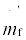and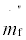were constants in time, similarly to tfi ' and tfi ''. We approached the periodic solution by going through enough cycles beginning with an arbitrary given distribution ts (x,0).

In the terminology of Schmidt and Willmott , this approach is an open method, as opposed to the closed method. The process began with the hot period taking the flow direction in the positive x-axis direction, so that the flow inflow boundary condition was given at x=0. The temperature distribution in solid material ts ' (x, P’), obtained at the end of the hot period, was used as the initial (or boundary) condition for the cold period. In reality, there is a short filling period between the hot and cold period (as well as between the cold and hot period) until the governing equations are valid once more. We ignored the filling period and started the cold period analysis immediately after τ=P'. Using dimensionless formulations, the computational situation is thus described schematically in Figure 3.

For a hot period, the governing dimensionless field equations are (see (1) and (2))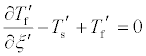(11)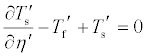(12)

with the boundary condition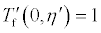(13)

Similarly, for a cold period the field equations are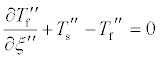(14)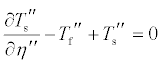(15)

with the boundary condition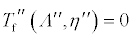(16)

Equation (14) shows a change of signs. Here ξ' and ξ'' are taken to grow in the same direction (Figure 3). However, mass flow rate mf '' is considered positive even when the flow direction is from right to left in the cold period. This is the reason for the change of signs in (14).

Using the dimensionless formulation, the initial (or boundary) conditions at the start of the hot and cold periods took the following forms, respectively,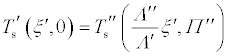(17)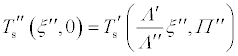(18)

The solution for each period was obtained by Formulation I, naturally taking into account the changes in signs in (14) and the righthand side boundary condition (16).

The code proceeded by solving the equations consecutively in hot and cold periods until error limit |δ' Λ|δ'' |<0.001 was reached. The error was checked at each period with previous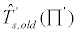and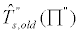and present value using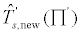and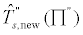values using expression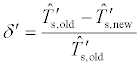(19)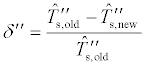(20)

The regenerator thermal ratios η’ REG and η'' REG described in  are of considerable final importance are of considerable final importance. Schmidt and Willmott  have described the thermal ratios as follows: “The effectiveness of regenerator behavior is measured in terms of the thermal ratio ηREG. This is defined to be the ratio of the actual heat transfer rate to the thermodynamically limited maximum obtainable heat transfer rate in a counterflow regenerator of infinite heat transfer area. Could this maximum rate be achieved, the temperature of the gas leaving the regenerator in hot/cold period would be equal to the entrance temperature.”

Expression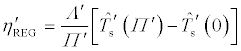(21)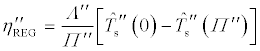(22)

can be derived. Here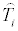is the mean dimensionless temperature (with respect to position) of the solid: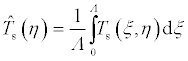(23)

We describe three example cases. For each case, we record similar calculation results.

The first example is a symmetrical case with the measures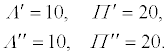(24)

The coarse, medium and fine meshes consisted of 4×8, 8×16 and 16×32 elements, respectively. Three total cycles were needed for “convergence” for each mesh density. The calculation results for the thermal ratios are given in Table 1.

Numerical solution in reference  Numerical solution (4 × 8) Numerical solution (8 × 16) Numerical solution
(16 × 32)
η'REG  0.494 0.49268 0.49332 0.49350
η"REG  0.494 0.49268 0.49332 0.49350

Table 1: Thermal ratio η'REG  and η"REG

It should be noted that the results from Schmidt and Willmott  (Table 5) were also obtained numerically. Temperature distributions in Ω are shown in Figure 4 and dimensional temperature distributions at τ=P' in Figure 5.

Figure 4: Fluid and solid dimensionless temperature distributions Tf and Ts (hot period on top line and cold period on bottom line, 4 × 8 coarse mesh).

Figure 5: (a) Dimensional fluid tf and (b) solid material ts temperature at t= P' with coarse, medium and fine meshes. Fluid hot inflow temperature tfi" = 10°C

Effective convergence rate r and relative (percentage) error estimate er calculated for η’ REG and η” REGare given in Table 2.

Hot period, η'REG  Cold period, η"REG
r 1.79863 1.79862
er 0.01874% 0.01874%

Table 2: Effective convergence rate for thermal ratios and relative (percentage) error estimates

The second example case is also taken from Schmidt and Willmott ; Example 5.5. The dimensionless measures are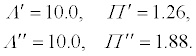(25)

The coarse, medium and fine meshes consisted of 8×4, 16×8 and 32×16 elements, respectively. Twenty five total cycles were needed for each mesh density. The calculation results for the thermal ratios are given in Table 3.

Numerical solution in referencea Numerical solution (25 × 4) Numerical solution (50 × 8) Numerical solution (100 × 16)
η'REG  0.947 0.96111 0.94956 0.94760
η"REG  0.635 0.64519 0.63746 0.63616

Table 3: Thermal ratio η'REG  and η"REG

Again, it should be noted that the results from (Schmidt and Willmott, (1981) were obtained numerically.

Temperature distributions in Ω are shown in Figure 6 and dimensional temperature distributions at τ=P' in Figure 7.

Figure 6: Fluid and solid dimensionless temperature distributions Tf and Ts (hot period on top line and cold period on bottom line, 25 × 4 coarse mesh).

Figure 7: (a) Solution domain in the dimensional x,Τ -space and (b) in the corresponding dimensionless ζ , η-space

Effective convergence rate r and relative (percentage) error estimate er calculated for η’ REG and η” REGare given in Table 4.

η'REG  η"REG
r 2.55493 2.57525
er 0.05317% 0.05138%

Table 4: Effective convergence rate for thermal ratios and relative (percentage) error estimates.

For the third example case, we slightly modified the first case so that the ratio was:

Λ'⁄Λ''= Π'⁄ Π''= 0.83333 and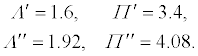(26)

The coarse, medium and fine meshes consisted of 4×8, 8×16 and 16×32 elements, respectively. Four total cycles were needed for each mesh density. The calculation results for the thermal ratios are presented in Table 5.

Numerical solution (4 × 8) Numerical solution (8 × 16) Numerical solution (16 × 32)
η'REG  0.36210 0.36271 0.36279
η"REG  0.36210 0.36271 0.36279

Table 5: Thermal ratio η'REG  and η"REG

Theory demands here that η' REG = η'' REG, which is seen to be satisfied by the numerical results. Temperature distributions in Ω are shown in Figure 8 and dimensional temperature distributions at τ=P' in Figure 9.

Figure 8: (a) Solution domain in the dimensional x,Τ -space and (b) in the corresponding dimensionless ζ , η-space

Figure 9: (a) Solution domain in the dimensional x,Τ -space and (b) in the corresponding dimensionless ζ , η-space

Effective convergence rate r and relative (percentage) error estimate er calculated for η’ REG and η” REGare given in Table 6.

η'REG  η"REG
r 2.84466 2.84835
er 0.00469% 0.00466%

Table 6: Effective convergence rate for thermal ratios and relative (percentage) error estimates.

As a general observation concerning Figures 4, 6 and 8, we may see the following. The dimensionless geometries in Figures 4 and 8 are rather similar and so are also the dimensionless temperature distributions. However, in the time direction narrow geometries of Figure 6 the temperature during the hot period “has not enough time” to penetrate far into the regenerator.

#### Conclusions

The results obtained in the three example cases presented above indicate that very good accuracy can be achieved with the finest meshes. The approach minimizes the effort of coding. It should not be difficult to extend the analysis to cases in which the inflow fluid temperature depends on time. This is achieved by simply changing the boundary conditions in the time direction appropriately. A more detailed presentation concerning the themes of the present article is given in Holopainen and Salonen .

The developed model can be applied to regenerators of both fixed and rotary heat storage mass. The model can be used as a tool in the design and optimization of heat exchangers when, for example, studying the effect of different hot and cold period lengths on outlet air temperatures and on thermal ratios.

#### Acknowledgements

The authors wish to thank Jorma Kinnunen, MSc (Tech.); Pasi Marttila, MSc (Tech.) and Jukka Tarvo, MSc (Tech.) for their advice on the use of COMSOL Multiphysics with MATLAB

#### References

Select your language of interest to view the total content in your interested language

### Article Usage

• Total views: 12436
• [From(publication date):
July-2015 - Aug 26, 2019]
• Breakdown by view type
• HTML page views : 8493
• PDF downloads : 3943

## Post your commentCan't read the image? click here to refresh
###### Peer Reviewed Journals

Make the best use of Scientific Research and information from our 700 + peer reviewed, Open Access Journals

International Conferences 2019-20

Meet Inspiring Speakers and Experts at our 3000+ Global Annual Meetings

Top